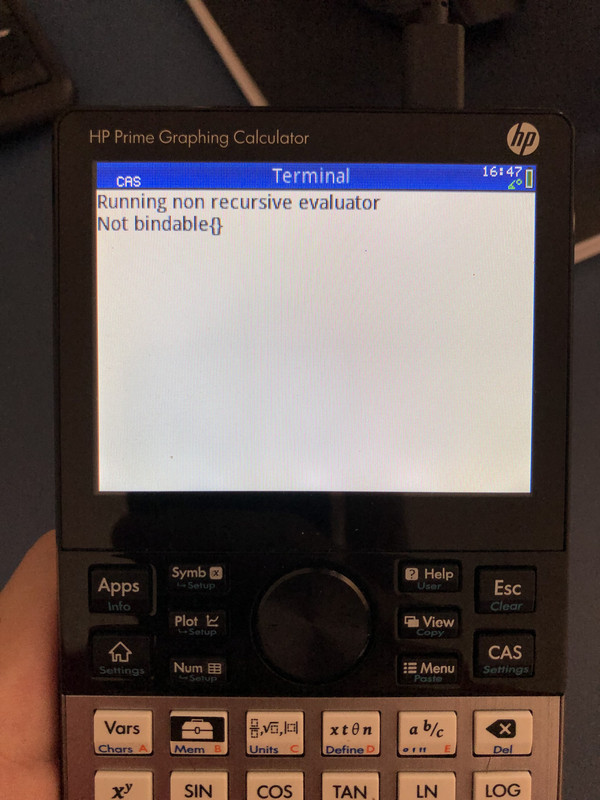# HP Forums

Full Version: Script to find the root locus
You're currently viewing a stripped down version of our content. View the full version with proper formatting.
The root locus part of the Control Systems program in this forum does not work.
I wrote a little script that can generate the root locus of a system, with instructions on how to plot.
With thanks to members @ThomasA and @Tim Wessman for suggestions on how to properly plot it.
Here is the code:

Code:
``` // This is a script to find the root locus of system // Arguments are num, den coefficients of polynomial // Stepp is the size of the change in K // Endd is the max value that K takes // Example:  rls([1,3],[1,7,14,8,0],0.01,10) // To use this program: // In the Statistics 2var, symbolic // Set independent varibale to L0 // Set dependent variable to L1 // Plot EXPORT rls(num, den, stepp, endd) BEGIN LOCAL begg; LOCAL xv:={}; LOCAL yv:={}; begg:=0.0001; FOR K FROM begg TO endd STEP stepp DO xv := concat(xv, RE(POLYROOT(poly2symb(den)+K*poly2symb(num)))); yv := concat(yv, IM(POLYROOT(poly2symb(den)+K*poly2symb(num)))); END; L0:= xv; L1 := yv; END;```
Inside CAS, point(proot(polynomial)) will display the list of roots of a polynomial. For several polynomials you can create an empty list l and run l:=concat(l,(proot(..)) inside the loop and run point(l) after the loop.
(11-29-2018 08:41 AM)parisse Wrote: [ -> ]Inside CAS, point(proot(polynomial)) will display the list of roots of a polynomial. For several polynomials you can create an empty list l and run l:=concat(l,(proot(..)) inside the loop and run point(l) after the loop.

That works better, thanks!

Code:
``` // This is a script to plot the root locus of a system // Arguments are num, den coefficients of polynomial // Stepp is the size of the change in K. If the script runs too slow, increase the stepp size.  // Endd is the max value that K takes, if it is too slow to plot, decrease the max value.  // Example:  rlscas([1,3],[1,7,14,8,0],0.01,10) #cas rlscas(num,den,stepp,endd):= BEGIN LOCAL K, begg, l:={}; begg:=0.001; FOR K FROM begg TO endd STEP stepp DO l := concat(l, proot(poly2symb(den)+K*poly2symb(num))); END; point(l); END; #end```
It surprisingly works on the PC emulator but not on the physical calculator!!

Error: Execute evaluator not recursive
Take a look what I've got on the calc:But in the emulator It runs nicely, I don't know why.
Reference URL's
• HP Forums: https://www.hpmuseum.org/forum/index.php
• :